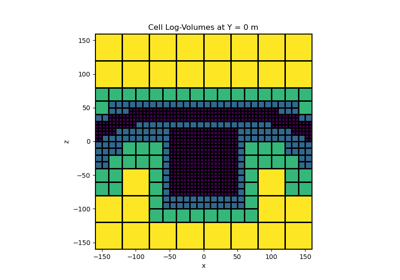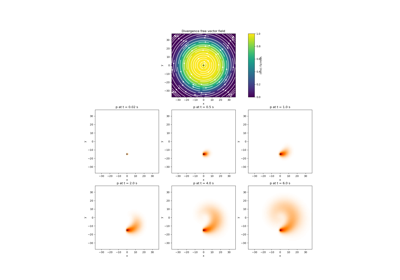# discretize.utils.mkvc¶

discretize.utils.mkvc(x, n_dims=1, **kwargs)[source]

Creates a vector with specified dimensionality.

This function converts a `numpy.ndarray` to a vector. In general, the output vector has a dimension of 1. However, the dimensionality can be specified if the user intends to carry out a dot product with a higher order array.

Parameters
xarray_like

An array that will be reorganized and output as a vector. The input array will be flattened on input in Fortran order.

n_dims`int`

The dimension of the output vector. `numpy.newaxis` are appened to the output array until it has this many axes.

Returns
`numpy.ndarray`

The output vector, with at least `n_dims` axes.

Examples

Here, we reorganize a simple 2D array as a vector and demonstrate the impact of the n_dim argument.

```>>> from discretize.utils import mkvc
>>> import numpy as np
```
```>>> a = np.random.rand(3, 2)
>>> a
array([[0.33534155, 0.25334363],
[0.07147884, 0.81080958],
[0.85892774, 0.74357806]])
```
```>>> v = mkvc(a)
>>> v
array([0.33534155, 0.07147884, 0.85892774, 0.25334363, 0.81080958,
0.74357806])
```

In Higher dimensions:

```>>> for ii in range(1, 4):
...     v = mkvc(a, ii)
...     print('Shape of output with n_dim =', ii, ': ', v.shape)
Shape of output with n_dim = 1 :  (6,)
Shape of output with n_dim = 2 :  (6, 1)
Shape of output with n_dim = 3 :  (6, 1, 1)
```

## Galleries and Tutorials using `discretize.utils.mkvc`¶